## Prove that the square of an odd number is always 1 more than a multiple of 4

Question

Prove that the square of an odd number is always 1 more than a multiple of 4

in progress 0
6 months 2021-07-25T20:54:32+00:00 1 Answers 16 views 0

## Answers ( )

By these examples you are able to see that the square of an odd number is always 1 more than a multiple of 4.

Step-by-step explanation:

For examples,

Let’s consider squares of 3, 11, 25, 37 and 131.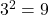8 is a multiple of 4, and 9 is more than 8.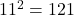120 is a multiple of 4 and 121 is one more than it.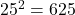624 is a multiple of 4 and 625 is one more than it.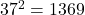1368 is a multiple of 4 and 1369 is one more than 1368.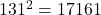17160 is a multiple of 4.